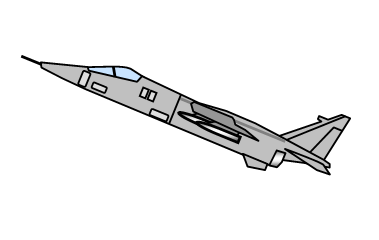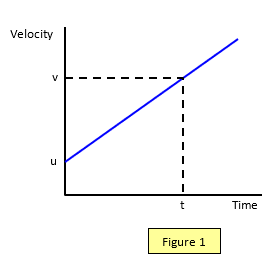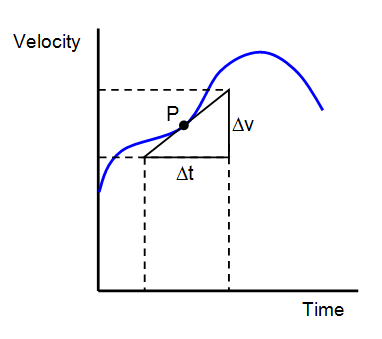# Motion with non-uniform velocityIf the velocity of an object changes its motion is called accelerated motion.

By referring to the section concerning Newton's laws of motion you might realise that the human body is not sensitive to velocity (if you shut your eyes!) but it can feel acceleration. The rate of acceleration is therefore of some importance in all transport systems.

It is also partly what determines how exciting (or frightening) a fairground ride is.
Acceleration, or better still, changing acceleration is a vital factor.

Our bodies experience a whole range of accelerations during our lives. A family car may reach 3 ms-2 during acceleration, the acceleration of objects falling freely in the Earth's gravitational field is about 9.8 ms-2 and pilots of military jets may reach up to 50 ms-2. The centripetal acceleration that we experience at latitude 51oN due to the rotation of the Earth is only 0.007 ms-2.

However some animals can experience large accelerations, a perch may reach 33 ms-2, a bush baby 180 ms- 2, a woodpecker 1000 ms-2 when pecking and when a flea jumps it may achieve 1400 ms-2!

Since velocity is a vector, acceleration can occur by the velocity either changing its size, its direction (as in a body going round a circle at a constant speed) or both. Acceleration is also a vector.

## Uniform acceleration

If the velocity is changing steadily the acceleration is given by the simple formula:

Acceleration (a) = [Final velocity (v) – Initial velocity (u)]/Time taken (t)
a = [v – u]/t
or
v = u + atThe units for acceleration are m/s2, at this level usually written as ms-2.

Uniform acceleration can be clearly shown by a velocity-time graph such as Figure 1.

Example problems
1. A car accelerates steadily from 12 ms-1 to 20 ms-1 in 4 s.
(a) what is the average speed of the car?
(b) what is the acceleration of the car?
(a) average speed = [20+12]/2 = 16 ms-1
(b) acceleration (a) = [20-12]/4 = 2 ms-2

2. A lorry brakes with an acceleration of –2.5 ms-2 from a speed of 18 ms-1 for 3s.
What is its velocity after the 3s of braking?
N.B Notice that the acceleration is given as negative because the lorry is slowing down.
Final velocity (v) = u + at = 18 + (-2.5x3) = 18 – 7.5 = 10.5 ms-1

## Non uniform acceleration

However if the acceleration is not uniform the more general definition must be used:

Acceleration (a) = rate of change of velocity = dv/dt = change in velocity/timeThe acceleration at a given time is therefore the gradient of the velocity-time curve at that time at the point P. (see Figure 2).

It is important to appreciate that the acceleration if the acceleration is not uniform then it is indeed the gradient and not simply the ratio of the value the velocity at that point to the time at that point.

Student investigation
Pilots and astronauts have to withstand large accelerations and decelerations. Find out the biological affects of such accelerations.

Student investigation
It is often stated in physics questions that the acceleration due to gravity is about 10 ms-2, but is it?
Devise and carry out two experiments based on different principles to measure the acceleration due to gravity.
Suggest where improvements may be made to improve the accuracy if you are not able to carry out the most accurate versions of your investigations.

A VERSION IN WORD IS AVAILABLE ON THE SCHOOLPHYSICS USB

© Keith Gibbs 2020